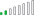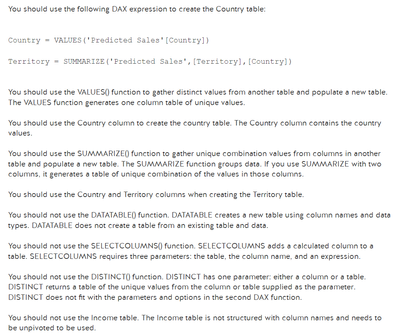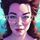cancel
Showing results for
Did you mean:

Fabric is Generally Available. Browse Fabric Presentations. Work towards your Fabric certification with the Cloud Skills Challenge.Frequent Visitor

## When to use Sum and Sumx

Hello All,

I have read so many articles but not able to understand when someone should use Sum and SumX , Average and AverageX.

Can someone explain with basic example  ?

Thanks

1 ACCEPTED SOLUTIONResolver II

SUM function: The SUM function calculates the sum of a specified column in a table. It takes a column or an expression that evaluates to a column as an argument.

SUM(<column>)

Example: Consider a table called "Sales" with columns "Product" and "SalesAmount." To calculate the total sales amount, you can use the SUM function as follows:

TotalSalesAmount = SUM(Sales[SalesAmount])

SUMX function: The SUMX function calculates the sum of an expression for each row in a table or an iterator. It iterates over each row and performs the specified calculation on the expression.

SUMX(<table>, <expression>)

Example: Suppose you have a table called "Orders" with columns "Product," "Quantity," and "Price." To calculate the total revenue for each product (quantity multiplied by price), you can use the SUMX function as follows:

TotalRevenue = SUMX(Orders, Orders[Quantity] * Orders[Price])

This will iterate over each row in the "Orders" table, calculate the revenue for each row (Quantity * Price), and then sum up the results to provide the total revenue.

Best Regards,

Ajith Prasath

If this post helps, then please consider Accept it as the solution to help the other members find it more quickly.

6 REPLIES 6Resolver II

SUM function: The SUM function calculates the sum of a specified column in a table. It takes a column or an expression that evaluates to a column as an argument.

SUM(<column>)

Example: Consider a table called "Sales" with columns "Product" and "SalesAmount." To calculate the total sales amount, you can use the SUM function as follows:

TotalSalesAmount = SUM(Sales[SalesAmount])

SUMX function: The SUMX function calculates the sum of an expression for each row in a table or an iterator. It iterates over each row and performs the specified calculation on the expression.

SUMX(<table>, <expression>)

Example: Suppose you have a table called "Orders" with columns "Product," "Quantity," and "Price." To calculate the total revenue for each product (quantity multiplied by price), you can use the SUMX function as follows:

TotalRevenue = SUMX(Orders, Orders[Quantity] * Orders[Price])

This will iterate over each row in the "Orders" table, calculate the revenue for each row (Quantity * Price), and then sum up the results to provide the total revenue.

Best Regards,

Ajith Prasath

If this post helps, then please consider Accept it as the solution to help the other members find it more quickly.Resolver II

SUM vs. SUMX:

• SUM: The SUM function is an aggregation function that calculates the sum of a column or expression within a single table or filter context. It works on a column and returns the sum of all the values in that column.
• SUMX: The SUMX function is an iterator function that allows you to perform a calculation (such as summing values) for each row of a table or an expression within a specific context. It works on an iterator table and applies the specified calculation to each row, and then aggregates the results.
• AVERAGE vs. AVERAGEX:
• AVERAGE: The AVERAGE function is an aggregation function that calculates the average of a column or expression within a single table or filter context. It works on a column and returns the average value of all the values in that column.
• AVERAGEX: The AVERAGEX function is an iterator function that allows you to perform a calculation (such as averaging values) for each row of a table or an expression within a specific context. It works on an iterator table and applies the specified calculation to each row, and then aggregates the results.Frequent Visitor

Hi Ajith,

Can you please explain with Example ?

Additionally I am facing dificultiies in generating Dax formulas how to practice those ?

For Ex. In the below screen shot I have used Distinct but the Asnwer is showing that we should use Summarize ? Which function to be used when how to understand those ?Super User

you can use the x aggregators when you need to get access to row context otherwise just use normal SUM, AVERAGE etc.Frequent Visitor

This reference guide gives some good examples: https://dax.guide/sumx/

First examples shows similarities:

--  SUM is the short version of SUMX, when used with one column only
--  SUMX is required to evaluate formulas, instead of columnsFrequent Visitor

I am facing dificultiies in generating Dax formulas how to practice those ?

For Ex. In the below screen shot I have used Distinct but the Asnwer is showing that we should use Summarize ? Which function to be used when how to understand those ?Announcements#### Power BI Monthly Update - November 2023

Check out the November 2023 Power BI update to learn about new features.#### Fabric Community News unified experience

Read the latest Fabric Community announcements, including updates on Power BI, Synapse, Data Factory and Data Activator.#### The largest Power BI and Fabric virtual conference

130+ sessions, 130+ speakers, Product managers, MVPs, and experts. All about Power BI and Fabric. Attend online or watch the recordings.Top Solution Authors
Top Kudoed Authors
Users online (4,010)